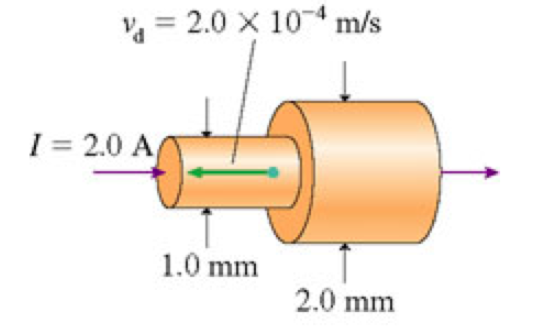# Problem: The two wires in the figure (Figure 1) are made of the same material. (a) What is the current in the 2.0-mm-diameter segment of the wire? (b) What is the electron drift speed in the 2.0-mm-diameter segment of the wire? (in μm/s)

###### FREE Expert Solution

Moment of inertia:

$\overline{){\mathbf{I}}{\mathbf{=}}{\mathbf{n}}{\mathbf{A}}{\mathbf{e}}{{\mathbf{v}}}_{{\mathbf{d}}}}$, where A is the area of the wire, vd is the drift speed.

A)

From the law of conservation of charge, the current that enters into the 1 mm segment of the wire is equal to the current in the 2mm segment of the wire.

84% (326 ratings)###### Problem Details

The two wires in the figure (Figure 1) are made of the same material.
(a) What is the current in the 2.0-mm-diameter segment of the wire?
(b) What is the electron drift speed in the 2.0-mm-diameter segment of the wire? (in μm/s)